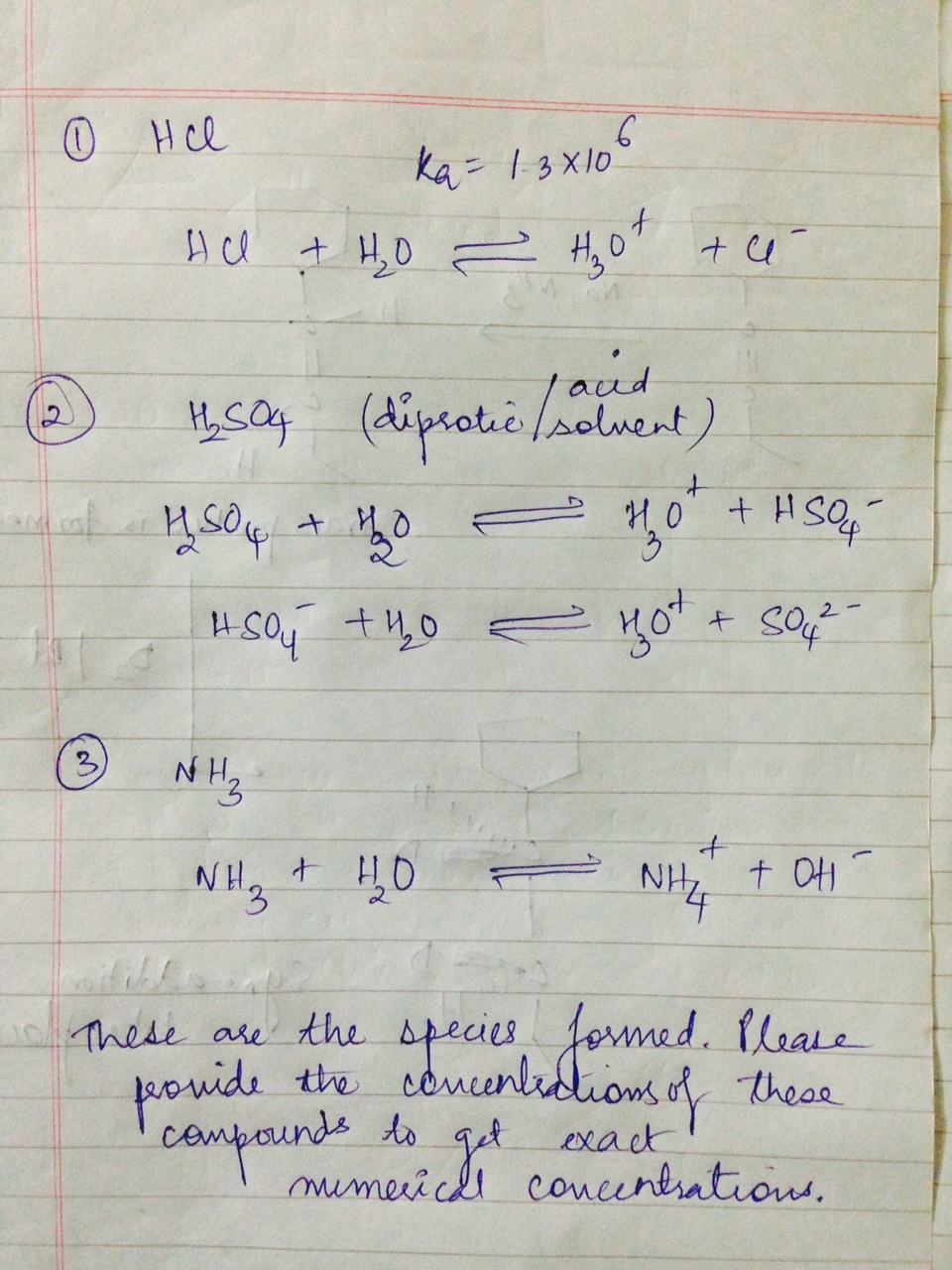Homework Help Question & Answers

# 1. Consult an appropriate source and determine the Concentration of each of the following species, when...

1. Consult an appropriate source and determine the Concentration of each of the following species, when in Concentrated form: HCl, H2SO4, and NH3.

2. A 10mL solution of 0.010M HCl is mixed with 20mL of a 0.01M Pb2+ solution, giving a total volume of 30mL. What are the concentrations of Cland Pb2+ after the mixing? Will a precipitate of PbCl2 form? (Ksp = 1.7 x 10-5 for PbCl2 at 25oC.)

3. In the Group 2 precipitations, the Sulfide Ion (S2- ) is generated in an Acidic environment. If the H2S concentration is maintained at 0.1M and the pH = 1, what is the [S2- ] concentration? (Ka1 = 1 x 10-7 and Ka2 = 1 x 10-13 for H2S.)

4. In the Group 3 precipitations, the pH is raised by adding Ammonia. Suppose it is raised to pH = 9. What is the [S2- ] concentration under these conditions? Assume the H2S concentration is again 0.1M.

#### Homework Answers

Answer #1Know the answer?
Your Answer:

#### Post as a guest

Your Name:

What's your source?

#### Earn Coin

Coins can be redeemed for fabulous gifts.

Not the answer you're looking for? Ask your own homework help question. Our experts will answer your question WITHIN MINUTES for Free.
Similar Homework Help Questions
• ### In the Group 3 precipitations, the pH is raised by adding Ammonia. Suppose it is raised...

In the Group 3 precipitations, the pH is raised by adding Ammonia. Suppose it is raised to pH = 9. What is the [S2- ] concentration under these conditions? Assume the H2S concentration is again 0.1M. Consult an appropriate source and determine the Concentration of each of the following species, when in Concentrated form: HCl, H2SO4, and NH3.

• ### 1. Using the given information answer the following questions with corresponding answers. (a) Tryptophan is a...

1. Using the given information answer the following questions with corresponding answers. (a) Tryptophan is a diprotic acid (Ka1 = 1.48 × 10-3 and Ka2 = 4.07 × 10-10) that can have three different form in solution: H2T+, HT, and T-. What would be the pH of the solution containing 0.045M HT? Group of answer choices 6.86 6.12 7.43 12.23 7.06 (b) Tryptophan is a diprotic acid (Ka1 = 1.48 × 10-3 and Ka2 = 4.07 × 10-10) that can...

• ### 1. Using the given information answer the following questions with corresponding answers. (a) Serine is a diprotic acid...

1. Using the given information answer the following questions with corresponding answers. (a) Serine is a diprotic acid (Ka1 = 6.17 × 10-3 and Ka2 = 7.08 × 10-10) that can have three different forms in solution: H2S+, HS, and S- (Note: S is not sulfur in these formulas). What would be the concentration of H2S+ in the solution of 0.105M Na+S-? Group of answer choices 8.29 × 10-8 M 1.62 × 10-12 M 9.62 × 10-9 M 4.15 ×...

• ### Help with any of these:    21

Help with any of these:    21. Aluminium sulphate is often used by gardeners to acidify soil and can be produced by the reaction 2Al(OH)3(aq) + 3 H2SO4(aq) à Al2(SO4)3(aq)  + 6 H2O(l) The volume of 0.275 mol/L H2SO4(aq) needed to react completely with 112 mL of 0.355 mol/l Al(OH)3(aq) is A. 96.4 mL B. 145 mL C. 168 mL D. 217 mL   22. To produce the anti cancer drug cisplatin (Pt (NH3)2Cl2(s)), a technician reacted NH3(aq) with excess K2PtCl4(aq). The...

Free Homework App

Scan Your Homework
to Get Instant Free Answers
Need Online Homework Help?

Get Answers For Free
Most questions answered within 3 hours.